Author: Oscar Cronquist Article last updated on August 20, 2021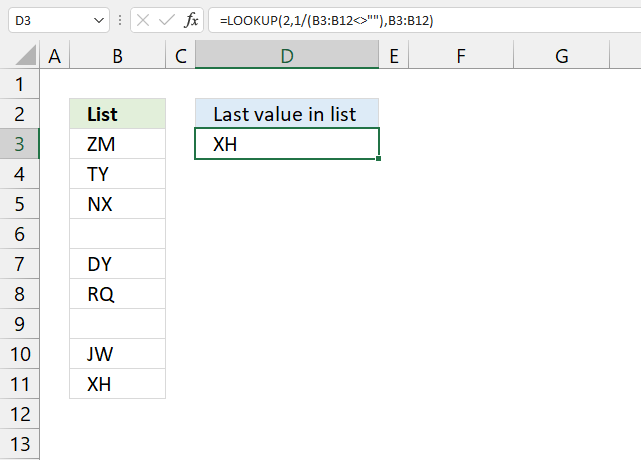This article demonstrates formulas that return the last value in a given cell range or column. The image above shows a formula in cell D3 that extracts the last value from cell range B3:B12.

Cell range B3:B12 is populated with values and empty cells in random order, this shows that the formula works fine with empty cells.

## 1. Extract the last value in a given column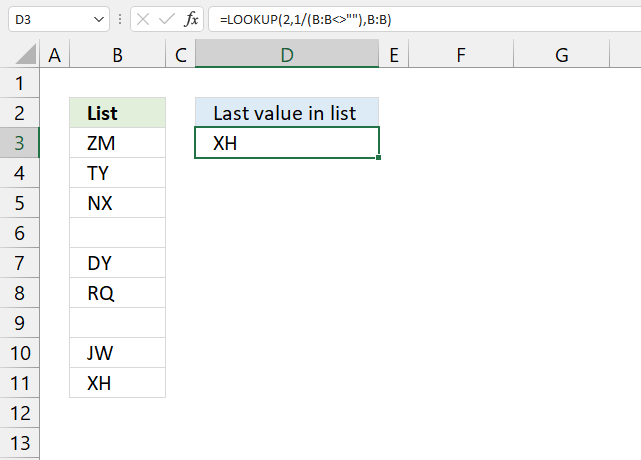The formula in cell D3 lets you get the last value in column B, it works fine with blank cells in your list.

Formula in cell D3:

=LOOKUP(2,1/(B:B<>""),B:B)

The formula is quite cpu-intensive since it is processing all cells in column B, there are more than a million cells in one column in Excel 2007 and later versions.

Recommended article

Recommended articles

Find last matching value in an unsorted list
This article demonstrates a formula that returns the last matching value based on a given condition. The above image shows […]

You can change the cell references if you know you will have a smaller list, this formula is easier for Excel to process:

=LOOKUP(2,1/(B1:B10000<>""),B1:B10000)

### 1.1 Explaining formula

Then it divides 1 with the array creating errors for all empty cells.

The LOOKUP function ignores errors and tries to find a match. If every match is 1 and the lookup value is 2, the LOOKUP function returns last value in cell range. Normally the list must be sorted ascending for the LOOKUP function to work, however since every value is 1 there is no need to sort the list.

I am going to use the following formula because it will be easier to demonstrate:

LOOKUP(2,1/(B3:B12<>""),B3:B12)

#### Step 1 - Check if cells in column B are not equal to nothing

The less than and greater than signs are logical operators, they check if cell values in column B are not empty. The result is an array containing TRUE or FALSE.

B3:B12<>""

becomes

{"ZM";"TY";"NX";0; "DY";"RQ";0; "JW";"XH";0}<>""

and returns

{TRUE;TRUE;TRUE; FALSE;TRUE;TRUE;FALSE; TRUE;TRUE;FALSE}

#### Step 2 - Divide 1 with array

1/(B3:B12<>"")

becomes

1/({TRUE;TRUE;TRUE; FALSE;TRUE;TRUE;FALSE; TRUE;TRUE;FALSE})

and returns

{1;1;1;#DIV/0!;1;1; #DIV/0!;1;1;#DIV/0!}

Boolean value TRUE is equal to 1 and FALSE is equal to 0. You can't divide a value with zero so Excel returns an error (#DIV/0!).

#### Step 3 - Return value

LOOKUP(2,1/(B3:B12<>""),B3:B12)

becomes

LOOKUP(2,{1;1;1;#DIV/0!;1;1; #DIV/0!;1;1;#DIV/0!},B3:B12)

becomes

LOOKUP(2,{1;1;1;#DIV/0!;1;1; #DIV/0!;1;1;#DIV/0!},{"ZM";"TY";"NX";0; "DY";"RQ";0; "JW";"XH";0})

and returns XH in cell D3.

Recommended article

Recommended articles

How to use the LOOKUP function
Finds a value in a sorted cell range and returns a value on the same row.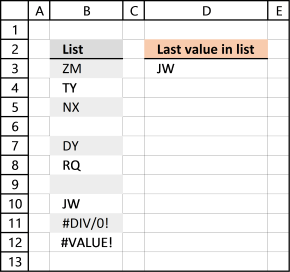I have added a few errors in column B, see the image above.

I am working with Excel 2016 and errors in column B seem to not be an issue.

## 2. Return a hyperlink to the last value in a column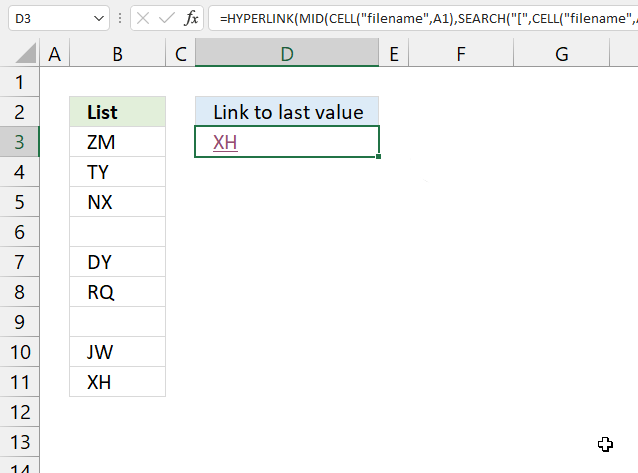The formula in cell D3, demonstrated in the image above, creates a hyperlink to the last cell in column B. Press with left mouse button on the hyperlink takes you to the last value in column B.

Formula in cell D3:

=HYPERLINK(MID(CELL("filename", A1), SEARCH("[", CELL("filename", A1)), LEN(CELL("filename", A1)))&"!\$B\$"&LOOKUP(2, 1/(B:B<>""), ROW(B:B)), LOOKUP(2, 1/(B:B<>""), B:B))

### 2.1 Explaining formula

#### Step 1 - Get path and workbook name

The CELL function gets information about the formatting, location, or the contents of a cell.

CELL("filename", A1)

returns C:\temp\[Find last value in listv2.xlsx]Hyperlink

#### Step 2 - Find the location of character [

We don't need the path in order to create a working hyperlink. This step returns the character of the first string that we do need.

The SEARCH function returns a number representing the position of character at which a specific text string is found reading left to right.

SEARCH("[", CELL("filename", A1))

becomes

SEARCH("[", "C:\temp\[Find last value in listv2.xlsx]Hyperlink")

and returns 35.

#### Step 3 - Extract workbook and worksheet name

The MID function returns a substring from a string based on the starting position and the number of characters you want to extract.

MID(text, start_num, num_chars)

MID(CELL("filename", A1), SEARCH("[", CELL("filename", A1)), LEN(CELL("filename", A1)))

becomes

MID(CELL("filename", A1), 9, LEN(CELL("filename", A1)))

The LEN function calculates the number of characters in a given string.

MID(CELL("filename", A1), 9, LEN(CELL("filename", A1)))

becomes

MID(CELL("filename", A1), 9, 49)

and returns

#### Step 4 - Concatenate workbook name, worksheet name, and column

The ampersand character & lets you concatenate strings in an Excel formula.

MID(CELL("filename", A1), SEARCH("[", CELL("filename", A1)), LEN(CELL("filename", A1)))&"!\$B\$"

becomes

and returns "[Find last value in listv2.xlsx]Hyperlink!\$B\$".

#### Step 5 - Calculate row number of the last vale in a column

The ROW function allows you to calculate the row number of the last value.

MID(CELL("filename", A1), SEARCH("[", CELL("filename", A1)), LEN(CELL("filename", A1)))&"!\$B\$"&LOOKUP(2, 1/(B:B<>""), ROW(B:B))

becomes

"[Find last value in listv2.xlsx]Hyperlink!\$B\$"&LOOKUP(2, 1/(B:B<>""), ROW(B:B))

becomes

and returns "[Find last value in listv2.xlsx]Hyperlink!\$B\$11"

#### Step 6 - Return the last value

LOOKUP(2, 1/(B:B<>""), B:B)

returns "XH".

#### Step 7 - Create a hyperlink

The HYPERLINK function allows you to build a link in a cell pointing to something else like a file, workbook, cell, cell range, or webpage.

HYPERLINK(MID(CELL("filename", A1), SEARCH("[", CELL("filename", A1)), LEN(CELL("filename", A1)))&"!\$B\$"&LOOKUP(2, 1/(B:B<>""), ROW(B:B)), LOOKUP(2, 1/(B:B<>""), B:B))

becomes

and returns a hyperlink to cell B11.

## 3. Return the row number of the last value in a column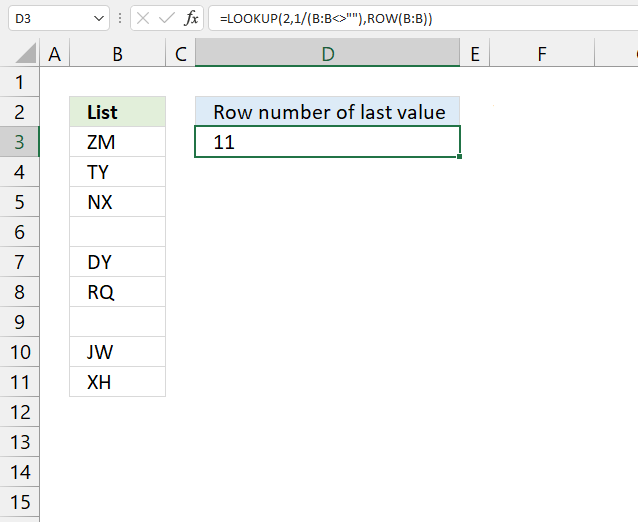The formula in cell D3 returns a number representing the row number of the last value in column B.

Formula in cell D3:

=LOOKUP(2, 1/(B:B<>""), ROW(B:B))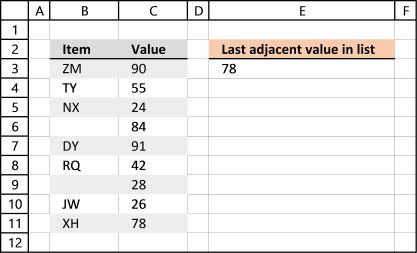This data set has two columns B and C. The formula in cell E3 returns the corresponding value of the last value in column B from column C.

Formula in cell E3:

=LOOKUP(2,1/(B:B<>""),C:C)

The formula returns an adjacent value of the last value. In fact, it doesn't need to be adjacent, you can change the cell reference (C:C) as long as its starting point and ending point are the same as the first cell reference (B:B).

## 5. Find the last non-empty cell with short cut keys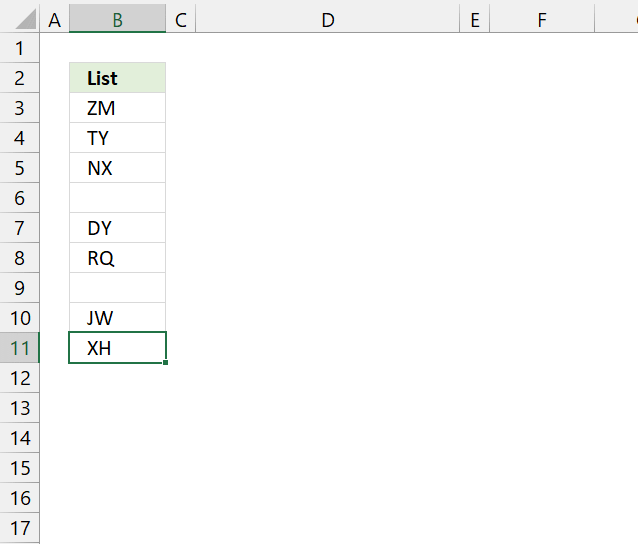The following steps decribe how to select the last non-empty cell in column B using keyboard keys.

1. Press with the left mouse button on cell A1 to select it.
2. Press and hold CTRL key.
3. Press down arrow key once. This takes you to the last cell in column A, if all cells in column A are empty.
4. Release all keys. Press right arrow key to move to the last cell in column B.
5. Press and hold CTRL key.
6. Press up arrow key once. This takes you to the last non-empty cell in column B. See the image above.

### Get the Excel fileFind-last-value-in-listv2.xlsx